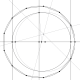# TEDx “Zeroes”

I spoke at TEDx University of Ljubljana. The topic was how programming influences various aspects of life. I showed the audence how a bit of simple programming can reveal the beauty of mathematics. Taking John Baez's The Bauty of Roots as an inspiration, I drew a very large image (20000 by 17500 pixels) of all roots of all polynomials of degree at most 26 whose coefficients are $-1$ or $1$. That's 268.435.452 polynomials and 6.979.321.752 roots. It is two degrees more than Sam Derbyshire's image,  so consider the race to be on! Who can give me 30 degrees?

### The code

The zeroes GitHub repository contains the code.

There really is not much to show. The C program which computes the zeroes uses the GNU Scientific Library routines for zero finding and is just 105 lines long. It generates a PPM image which I then processed with ImageMagick and ffmpeg. The real work was in the image processing and the composition of movies. I wrote a helper Python program that lets me create floyovers the big image, and I became somewhat of an expert in the use of ImageMagick.

The code also contains a Python program for generating a variation of the picture in which roots of lower degrees are represented by big circles. I did not show any of this in the TEDx talk but it is available on Github.

Oh, and a piece of Mathematica code that generates the zeroes fits into a tweet.

### The videos

The “Zeroes” YouTube channel contains the animations. The ones I showed in the TEDx talk are in Full HD (1920 by 1080). There is also a lower resolution animation of how zeroes move around when we change the coefficients. Here is one of the movies, but you really should watch it in Full HD to see all the details.

### The pictures

• The computed image zeroes.ppm.gz (125 MB) at 20000 by 17500 pixels is stored in the PPM format. The picture is dull gray, and is not meant to be viewed directly.
• The official image zeroes26.png (287 MB) at 20000 by 175000 pixels in orange tones. Beware,  it can bring an image viewing program to its knees.
• I computed tons of closeups to generate the movies. Here are the beginnings of each animation available at Vimeo, and measly 1920 by 1080 pixels each (click on them).One feature of the pictures in John Baez's post is a grey line on the real line due to the tendency of polynomials to have real-valued roots. However, I notice your picture doesn't have that. Why is that?Because I deleted the line. This is known as "artistic freedom".kram1032

I think there might be a more natural choice for selecting polynomial coefficients as points. It actually came to me while reading through some of the answers you got on MathOverflow. I wonder if this choice would reveal anything different.

As you know you can construct polynomials of degree n simply by multiplying together a bunch of monomials with similar degrees. If you do this with generic elements you'll get: 1) x - x0 2) x² - (x0 + x1) x + x0 x1 3) x³ - (x0 + x1 + x2) x² + (x0 x1 + x0 x2 + x1 x2) x - x0 x1 x2 ... Notice first of all, that the order of the linear combinations of the summed terms increase as the power decreases, so, for instance, for the 3rd degree case:

r? x³ - r x² + r² x - r³

But there is more structure here: The number of terms follows the binomial coefficients. So really it should be: x³ - 3 r x² + 3 r² x - r³

So it seems like a natural choice to me to cut off sequences by that rule: Pick an r. Calculate the roots of all polynomials of order n where the ith coefficient ranges over (- binomial(n, i)r^(n-i), binomial(n, i)r^(n-i)).

The only thing I'm not quite sure about is what to do with polynomials where the top coefficient isn't 1. One choice might be to just let it range over (1, r) (noting that the sign change won't matter regardless; alternatively, if you want to consider roots of lower-degree polynomials, also allow for 0). Equivalently you could also just allow fractional coefficients for a given maximum choice of denominator. I'm not sure if anything more natural exists.

I wonder how his might affect the picture.On the one hand, the algebraics are dense (even the algebraic integers are dense) in the complex plane... so one of your pictures would fill in closer around easy roots and have its outer edges much further away, and have a lot more stuff in the middle... I'm expecting it'd be fuzzier, too; there are reasonable algorithms taking two polynomials and returning a polynomial whose roots are sums of the given polynomials' roots: trying to enumerate all polynomials means you're eventually asking for your root-set to be an approximate-group in the sense that (e.g.) Terry Tao writes about...

AND it would take a LOT longer to list all the polynomials you're aksing for roots of! Even for just the polynomials of degree n with coefficients in [-n,...,n], there are well more than n^n of them!Were there not some pictures of all algebraic numbers? Here it is: http://blogs.ams.org/visualinsight/2013/09/01/algebraic-numbers/A Lovely Picture! But The text there explains further "the color of a point indicates the degree of the polynomial of which it’s a root" and "The size of a point decreases exponentially with the ‘complexity’ of [its minimal polynomial]"; which sounds like a rather different highlighting scheme than asking for the raw density of roots around some point.

Write your comment using Markdown. Use $⋯$ for inline and $$⋯$$ for display LaTeX formulas, and <pre>⋯</pre> for display code. Your E-mail address is only used to compute your Gravatar and is not stored anywhere. Comments are moderated through pull requests.